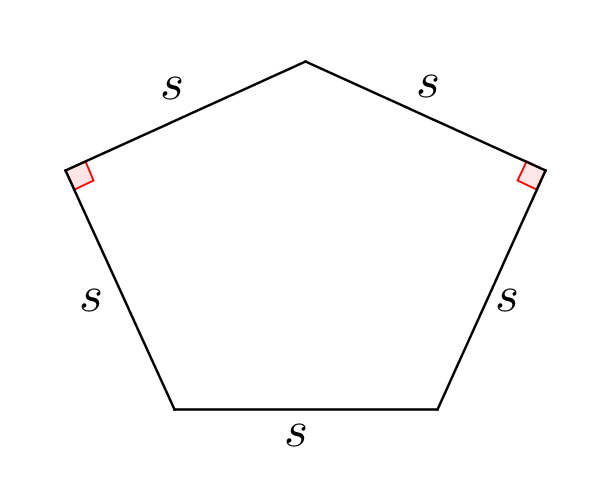# Similar Pentagon Areas Part 2

Geometry Level 2

Let a pentasquish be a pentagon with the following properties:If the area of a pentasquish is $\left(a+\dfrac{\sqrt{b}}{c} \right)s^2$, where $s$ is the length of one of the sides, and $a$, $b$, and $c$ are positive integers with $b$ square-free, find $a+b+c$.